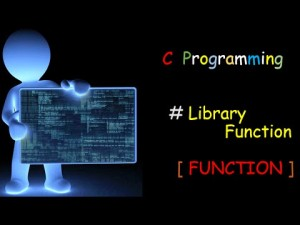3. How can I write functions that take a variable number of arguments?

Use <stdarg.h>. This defines some macros that let your program deal with variable numbers of arguments.

There’s no portable way for a C function, with no constraints on what it might be passed, to know how many arguments it might have gotten or what their types are. If a C function doesn’t take a fixed number of arguments (of fixed types), it needs some convention for what the arguments are. For example, the first argument to printf is a string, which indicates what the remaining arguments are:

printf(“Hello, world!\n”);   /* no more arguments */

printf(“%s\n”, “Hello, world!”);   /* one more string argument */

printf(“%s, %s\n”, “Hello”, “world!”);   /* two more string arguments */

printf(“%s, %d\n”, “Hello”, 42);   /* one string, one int */

The below program shows a simple printf-like function. The first argument is the format; from the format string, the number and types of the remaining arguments can be determined. As with the real printf, if the format doesn’t match the rest of the arguments, the result is undefined. There’s no telling what your program will do then (but probably something bad).

#include  <stdio.h>

#include    <stdlib.h>

#include    <string.h>

#include    <stdarg.h>

static char * int2str(int n)

{

int minus = (n < 0);

static char     buf;

char    *p = &buf;

if (minus)

n = -n;

*p = ‘\0’;

do {

*–p = ‘0’ + n % 10;

n /= 10;

} while (n > 0);

if (minus)

*–p = ‘-‘;

return p;

}

/* This is a simple printf-like function that handles only

the format specifiers %%, %s, and %d. */

void simplePrintf(const char *format, …)

{

va_list ap; /* ap is our argument pointer. */

int     i;

char    *s;

va_start(ap, format);

for ( ; *format; format++)

{

if (*format != ‘%’)

{

putchar(*format);

continue;

}

switch (*++format)

{

case ‘s’:

/* Get next argument (a char*) */

s = va_arg(ap, char *);

fputs(s, stdout);

break;

case ‘d’:

/* Get next argument (an int) */

i = va_arg(ap, int);

s = int2str(i);

fputs(s, stdout);

break;

case ‘\0’:

format–;

break;

default:

putchar(*format);

break;

}

}

/* Clean up varying arguments before returning */

va_end(ap);

}

void main()

{

simplePrintf(“The %s tax rate is %d%%.\n”, “sales”, 6);

}

3. How can I write functions that take a variable number of arguments?

Use <stdarg.h>. This defines some macros that let your program deal with variable numbers of arguments.

There’s no portable way for a C function, with no constraints on what it might be passed, to know how many arguments it might have gotten or what their types are. If a C function doesn’t take a fixed number of arguments (of fixed types), it needs some convention for what the arguments are. For example, the first argument to printf is a string, which indicates what the remaining arguments are:

printf(“Hello, world!\n”);   /* no more arguments */

printf(“%s\n”, “Hello, world!”);   /* one more string argument */

printf(“%s, %s\n”, “Hello”, “world!”);   /* two more string arguments */

printf(“%s, %d\n”, “Hello”, 42);   /* one string, one int */

#include  <stdio.h>

#include    <stdlib.h>

#include    <string.h>

#include    <stdarg.h>

static char * int2str(int n)

{

int minus = (n < 0);

static char     buf;

char    *p = &buf;

if (minus)

n = -n;

*p = ‘\0’;

do {

*–p = ‘0’ + n % 10;

n /= 10;

} while (n > 0);

if (minus)

*–p = ‘-‘;

return p;

}

/* This is a simple printf-like function that handles only

the format specifiers %%, %s, and %d. */

void simplePrintf(const char *format, …)

{

va_list ap; /* ap is our argument pointer. */

int     i;

char    *s;

va_start(ap, format);

for ( ; *format; format++)

{

if (*format != ‘%’)

{

putchar(*format);

continue;

}

switch (*++format)

{

case ‘s’:

/* Get next argument (a char*) */

s = va_arg(ap, char *);

fputs(s, stdout);

break;

case ‘d’:

/* Get next argument (an int) */

i = va_arg(ap, int);

s = int2str(i);

fputs(s, stdout);

break;

case ‘\0’:

format–;

break;

default:

putchar(*format);

break;

}

}

/* Clean up varying arguments before returning */

va_end(ap);

}

void main()

{

simplePrintf(“The %s tax rate is %d%%.\n”, “sales”, 6);

}